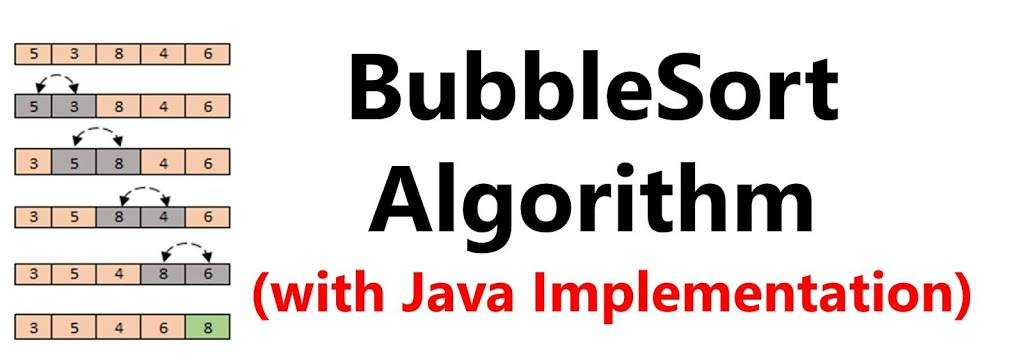# How Bubble Sort Algorithm Works (Implementation in Java)This would be a very simple explanation with the program in Java on how the Bubble Sort Algorithms works.

Bubble Sort works by iterating through the elements of the array and doing pairwise swap of adjacent elements that are out of order.

We would examine the program in Java and then explain it line by line. The we would actually run the program using Netbeans IDE to see how it works.

The java program is explained as follows: There would be three functions in addition to the main methods

BubbleSort(): This is the function that performs the sorting on an array. This function takes an array as parameter.

```    //THE BUBBLESORT FUNCTION IN JAVA
public static void BubbleSort(int[] a)
{
int i, j;
int N = a.length;```

for(j=N1; j>0; j–) {
for(i=0; i<j; i++){
if(a[i]> a[i+1])
swap(a, i, i+1);
}
}
}

Listing 1: BubbleSort function

Swap(): This function performs a pairwise swap of two array elements. It take three parameters: the first is the array, the second and third are the indices of the elements to be swapped.

```    //FUNCTION TO PERFORM SWAP OF TWO ITEMS
public static void swap(int[] ar, int x, int y)
{
int k = ar[x];
ar[x] = ar[y];
ar[y] = k;
}```

Listing 2: Swap Function

Display(): This function displays the elements of the array to the output by looping through the array elements and calling System.out.println().

```    //FUNCTION TO DISPLAY ARRAY ITEMS TO THE OUTPUT
public static void Display(int a[])
{
int i=0;
while(i != a.length)
{
System.out.println(a[i]);
i++;
}
}```

Listing 3: Swap Function

Main(): Runs the program by calling the each of the functions.

```    //MAIN PROGRAM
public static void main(String[] args) {
int a[] = {5,7,3,8,0,2};    //INITIALIZE AN ARRAY WE ARE GOING TO SORT
Display(a);                 //DISPLAY THE UNSORTED ARRAY
BubbleSort(a);              //SORT THE ARRAY USING BUBBLESORT
System.out.println("The Sorted list is given below:");
Display(a);                 //DISPLAY THE SORTED ARRAY
}```

Listing 4: Main Program

This short video explains how to put everything together and run it in Netbeans